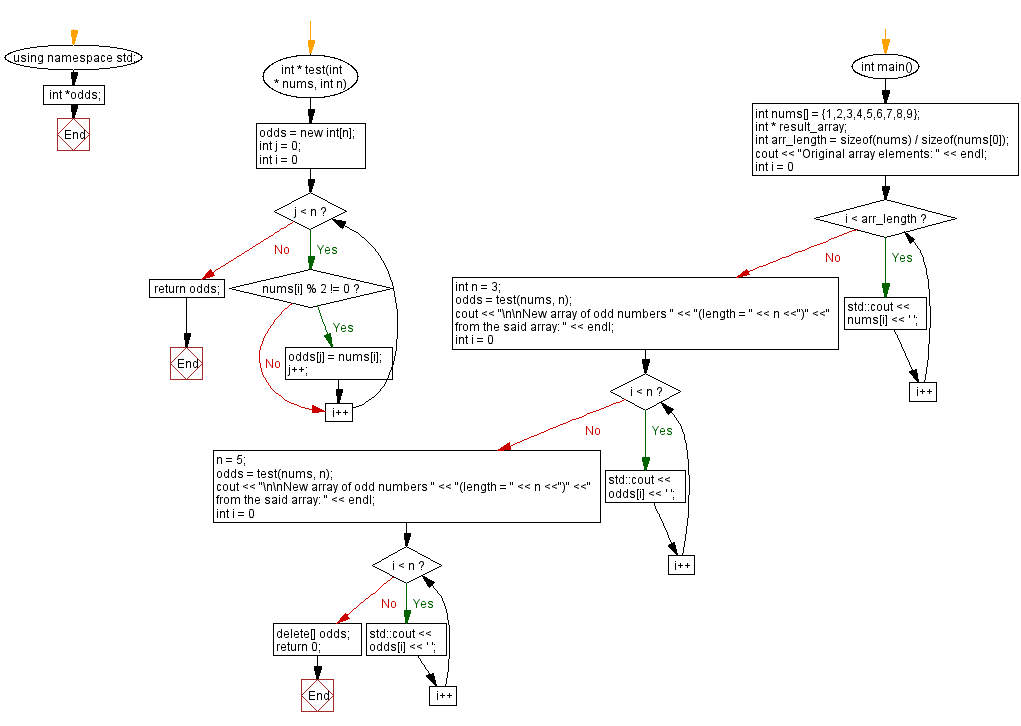﻿ CPP - Create an array of odd numbers from an array

# C++ Exercises: Create an array of odd numbers and specific lengths from an array

## C++ Basic Algorithm: Exercise-123 with Solution

Write a C++ program that creates a new array of odd numbers with specific lengths from a given array of positive integers.

Test Data:
({1,2,3,5,7,9,10},3) -> {1,3,5}

Sample Solution:

C++ Code :

``````#include <iostream>
#include <string.h>
using namespace std;
int *odds;

int * test(int * nums, int n) {
odds = new int[n];
int j = 0;

for (int i = 0; j < n; i++)
{
if (nums[i] % 2 != 0)
{
odds[j] = nums[i];
j++;
}
}

return odds;
}

int main() {

int nums[] = {1,2,3,4,5,6,7,8,9};
int * result_array;
int arr_length = sizeof(nums) / sizeof(nums);
cout << "Original array elements: " << endl;
for (int i = 0; i < arr_length; i++) {
std::cout << nums[i] << ' ';
}
int n = 3;
odds = test(nums, n);
cout << "\n\nNew array of odd numbers " << "(length = " << n <<")" <<" from the said array: " << endl;
for (int i = 0; i < n; i++) {
std::cout << odds[i] << ' ';
}
n = 5;
odds = test(nums, n);
cout << "\n\nNew array of odd numbers " << "(length = " << n <<")" <<" from the said array: " << endl;
for (int i = 0; i < n; i++) {
std::cout << odds[i] << ' ';
}
delete[] odds;
return 0;
}

``````

Sample Output:

```Original array elements:
1 2 3 4 5 6 7 8 9

New array of odd numbers (length = 3) from the said array:
1 3 5

New array of odd numbers (length = 5) from the said array:
1 3 5 7 9

```

Flowchart:C++ Code Editor:

What is the difficulty level of this exercise?

﻿

## C++ Programming: Tips of the Day

Why is there no std::stou?

The most pat answer would be that the C library has no corresponding "strtou", and the C++11 string functions are all just thinly veiled wrappers around the C library functions: The std::sto* functions mirror strto*, and the std::to_string functions use sprintf.

Ref: https://bit.ly/3wtz2qA

We are closing our Disqus commenting system for some maintenanace issues. You may write to us at reach[at]yahoo[dot]com or visit us at Facebook